### Question 5. A stone thrown vertically upwards with initial velocity u reaches a height ‘h’ before coming down. Show that the time taken to go up is same as the time taken to come down.

Question 5.

A stone thrown vertically upwards with initial velocity u reaches a height ‘h’ before coming down. Show that the time taken to go up is same as the time taken to come down.

Given;

Initial velocity = u;

Distance travelled(s) = h;

Diagram below shows the situation given in the question;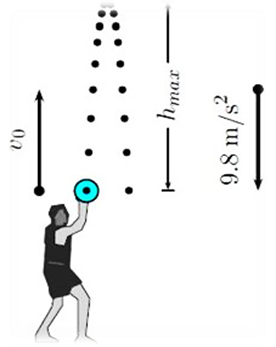Time to go up (t1): By Newton’s first equation of the motion

v = u + at

Where v = final velocity;

u= initial velocity

a = acceleration;

t = time taken;

According to our question;

v = 0 (because object has reach a maximum height ‘h’ before coming down and velocity at maximum height is zero);

u= u;

a = -g (because when object will be going up the acceleration due to gravity will be acting downwards to make object to fall. Hence by sign convention direction of motion and acceleration is opposite therefore a is negative);

T = t1;

Putting the values in the equation of motion we get;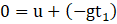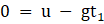.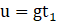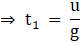Time of descent (t2): By Newton’s second equation of motion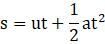Where;

s = Distance travelled;

u = initial velocity;

a = acceleration;

t = time taken;

According to our question;

s = h;

u = 0 (When the object is at maximum height its velocity is zero);

a = g (because when object will be down the acceleration due to gravity will be acting downwards to make object to fall. Hence by sign convention direction of motion and acceleration is same therefore a is positive);

T = t2;

Putting the values in the equation we get;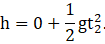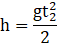From newton’s third equation of the motion;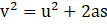Where symbols have usual meanings as above;

When the object is descending down

u = 0 (When the object is at maximum height its velocity is zero);

v = u (Final velocity will be the same as initial velocity);

a = g (because when object will be down the acceleration due to gravity will be acting downwards to make object to fall. Hence by sign convention direction of motion and acceleration is same therefore a is positive);

s =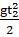;

Putting values in the equation of motion ;

Hence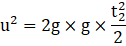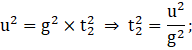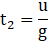Since t1 = t2 =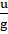Hence the time of ascent and time of descent are equal;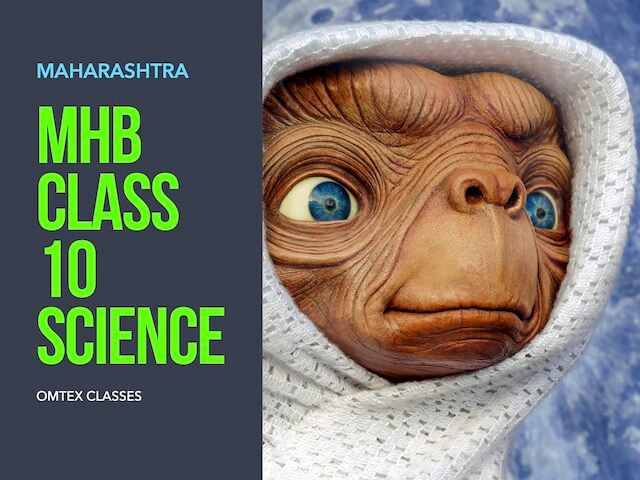## PDF FILE TO YOUR EMAIL IMMEDIATELY PURCHASE NOTES & PAPER SOLUTION. @ Rs. 50/- each (GST extra)

SUBJECTS

HINDI ENTIRE PAPER SOLUTION

MARATHI PAPER SOLUTION
SSC MATHS I PAPER SOLUTION
SSC MATHS II PAPER SOLUTION
SSC SCIENCE I PAPER SOLUTION
SSC SCIENCE II PAPER SOLUTION
SSC ENGLISH PAPER SOLUTION
SSC & HSC ENGLISH WRITING SKILL
HSC ACCOUNTS NOTES
HSC OCM NOTES
HSC ECONOMICS NOTES
HSC SECRETARIAL PRACTICE NOTES

2019 Board Paper Solution

HSC ENGLISH SET A 2019 21st February, 2019

HSC ENGLISH SET B 2019 21st February, 2019

HSC ENGLISH SET C 2019 21st February, 2019

HSC ENGLISH SET D 2019 21st February, 2019

SECRETARIAL PRACTICE (S.P) 2019 25th February, 2019

HSC XII PHYSICS 2019 25th February, 2019

CHEMISTRY XII HSC SOLUTION 27th, February, 2019

OCM PAPER SOLUTION 2019 27th, February, 2019

HSC MATHS PAPER SOLUTION COMMERCE, 2nd March, 2019

HSC MATHS PAPER SOLUTION SCIENCE 2nd, March, 2019

SSC ENGLISH STD 10 5TH MARCH, 2019.

HSC XII ACCOUNTS 2019 6th March, 2019

HSC XII BIOLOGY 2019 6TH March, 2019

HSC XII ECONOMICS 9Th March 2019

SSC Maths I March 2019 Solution 10th Standard11th, March, 2019

SSC MATHS II MARCH 2019 SOLUTION 10TH STD.13th March, 2019

SSC SCIENCE I MARCH 2019 SOLUTION 10TH STD. 15th March, 2019.

SSC SCIENCE II MARCH 2019 SOLUTION 10TH STD. 18th March, 2019.

SSC SOCIAL SCIENCE I MARCH 2019 SOLUTION20th March, 2019

SSC SOCIAL SCIENCE II MARCH 2019 SOLUTION, 22nd March, 2019

XII CBSE - BOARD - MARCH - 2019 ENGLISH - QP + SOLUTIONS, 2nd March, 2019

HSC Maharashtra Board Papers 2020

(Std 12th English Medium)

HSC ECONOMICS MARCH 2020

HSC OCM MARCH 2020

HSC ACCOUNTS MARCH 2020

HSC S.P. MARCH 2020

HSC ENGLISH MARCH 2020

HSC HINDI MARCH 2020

HSC MARATHI MARCH 2020

HSC MATHS MARCH 2020

SSC Maharashtra Board Papers 2020

(Std 10th English Medium)

English MARCH 2020

HindI MARCH 2020

Hindi (Composite) MARCH 2020

Marathi MARCH 2020

Mathematics (Paper 1) MARCH 2020

Mathematics (Paper 2) MARCH 2020

Sanskrit MARCH 2020

Important-formula

THANKS